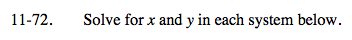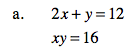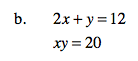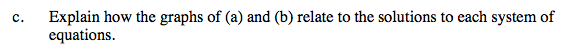### Home > A2C > Chapter 11 > Lesson 11.2.3 > Problem11-72

11-72.Solve for y in one of the equations and substitute.

y = 12 − 2x

$y=\frac{16}{x}$

(2, 8) and (4, 4)See part (a).

(3 + i, 6 − 2i) and (3 − i, 6 + 2i)What does it mean if the solutions contain i?